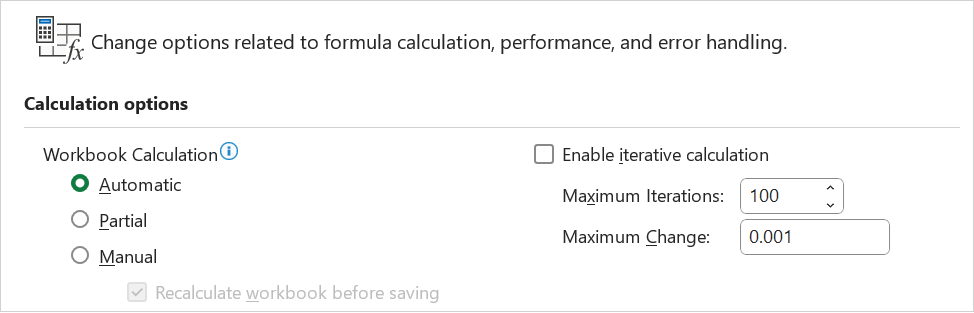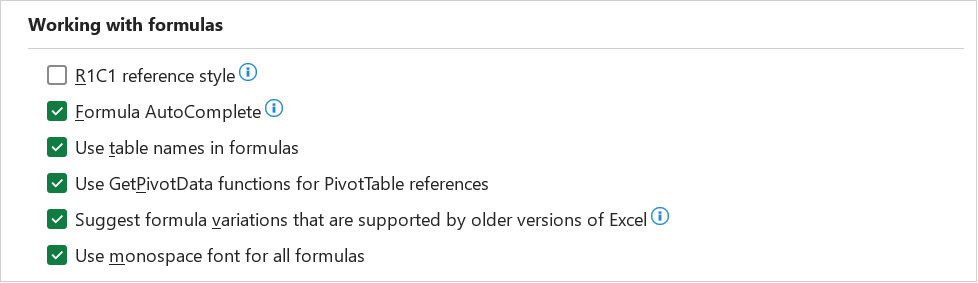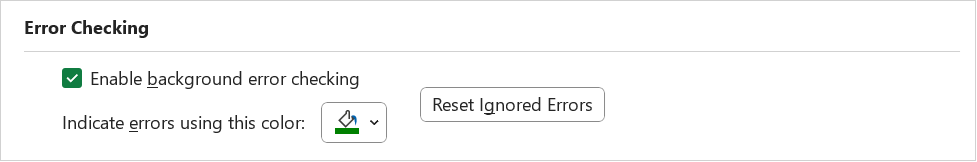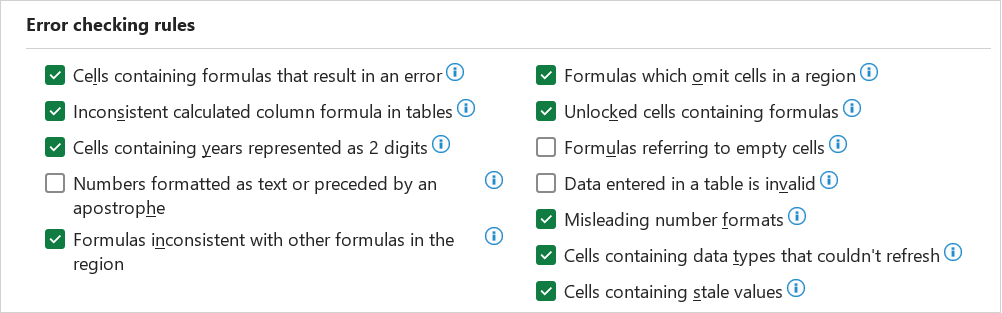### Formulas

#### Calculation optionsAutomatic - (Application Setting) Calculates all dependent formulas every time you make a change to a value, formula or name. Any workbook that contains formulas will be automatically calculated when it is opened. This is the default calculation setting. more
Automatic except tables - Calculates all dependent formulas except those in data tables. Data tables will only be recalculated when one of its values are changed. You can calculate the formulas in data tables by pressing the "Calc Now" button.
Manual - Calculates only when requested by the user. more
Recalculate before save - Calculates all dependent formulas in the worksheet before it is saved.
Enable iteration calculation - This can be used to restrict the iteration when using circular references. more
Maximum iterations - This is the maximum number of iterations used before iteration will stop. The default is 100.
Maximum change - The largest number that the change in value, must be less than, before iteration will stop. The smaller the number the more accurate the result. The default is 0.001.

#### Working with formulasR1C1 reference style - Changes the reference style of row and column headings and cell references from A1 style to R1C1 style. In A1 style, columns are labeled alphabetically, and rows are labeled numerically. In R1C1 reference style, both columns and rows are labeled numerically.
Formula AutoComplete - Selected by default, this option lets you use Formula AutoComplete to easily create and edit formulas and to minimize typing and syntax errors. When you type an = (equal sign) to start a formula, followed by the beginning letters or a display trigger, Formula AutoComplete provides a dynamic drop-down list of valid functions, names, and text strings that match the letters or trigger so that you can simply insert an item in the drop-down list into the formula. Clear the Formula AutoComplete check box if you don't want to use Formula AutoComplete.
Use table names in formulas - Selected by default, this option makes it easier and more intuitive to work with table data when you use formulas that reference a table, either portions of a table or the entire table. Clear the Use table names in formulas check box if you don't want to use table names in formulas.
Use GetPivotTable functions for PivotTable references - Determines the type of cell reference that is created for a PivotTable cell when you use semi-selection in a formula outside of the PivotTable. Select the check box to create a GETPIVOTDATA function cell reference, such as GETPIVOTDATA("Sales",\$A\$4). Clear the check box to create an A1 reference style cell reference, such as A4.

#### Error checkingEnable background error checking - Select to have Excel check cells to for errors at idle. If a cell is found to have an error, the cell is flagged with an indicator in the upper left corner of the cell.
Indicate errors using this color - Sets the color that Excel uses to indicate errors. If you click Automatic, the color is set to the default color of green.
Reset Ignored Errors - Click to flag errors in the spreadsheet and to find them when checking for errors even though they have already been found and ignored.

#### Error checking rulesCells containing formulas that result in an error - Select to have Excel treat cells that contain formulas that result in an error as an error and to display a warning.
Inconsistent calculated column formula in tables - Select to have Excel treat cells that contain formulas or values that are inconsistent with the column formula or tables as an error and to display a warning. This option refers to Calculated Columns.
Cells containing years represented as 2 digits - Select to have Excel treat formulas that contain text-formatted cells with years represented as 2 digits as an error and to display a warning when checking for errors.
Numbers formatted as text or preceded by an apostrophe - Select to have Excel treat numbers formatted as text or preceded by an apostrophe as an error and to display a warning.
Formulas inconsistent with other formulas in the region - Select to have Excel treat a formula in a region of your worksheet that differs from the other formulas in the same region as an error and to display a warning.
Formulas which omit cells in a region - Select to have Excel treat formulas that omit certain cells in a region as an error and to display a warning.
Unlocked cells containing formulas - Select to have Excel treat an unlocked cell that contains a formula as an error and to display a warning when checking for errors.
Formulas referring to empty cells - Select to have Excel treat formulas that refer to empty cells as an error and to display a warning.
Data entered in a table is invalid - Select to have Excel treat cells that contain values that are inconsistent with the column data type for tables that are connected to data in a SharePoint list as an error and to display a warning.